## Matrix Norm

Given a Square Matrixwith Complex (or Real) entries, a Matrix Normis a Nonnegative number associated withhaving the properties

1.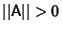whenand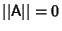Iff,

2.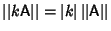for any Scalar,

3.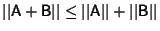,

4.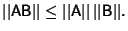For anMatrixand anUnitary Matrix,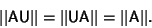Let, ...,be the Eigenvalues of, thenThe Maximum Absolute Column Sum Norm, Spectral Norm, and Maximum Absolute Row Sum NormsatisfyFor a Square Matrix, the Spectral Norm, which is the Square Root of the maximum Eigenvalue of(where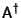is the Adjoint Matrix), is often referred to as the'' matrix norm.

See also Compatible, Hilbert-Schmidt Norm, Maximum Absolute Column Sum Norm, Maximum Absolute Row Sum Norm, Natural Norm, Norm, Polynomial Norm, Spectral Norm, Spectral Radius, Vector Norm

References

Gradshteyn, I. S. and Ryzhik, I. M. Tables of Integrals, Series, and Products, 5th ed. San Diego, CA: Academic Press, pp. 1114-1125, 1979.